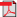RIEB Discussion Paper Series No.2016-05Title

Infinite-Horizon Deterministic Dynamic Programming in Discrete Time: A Monotone Convergence Principle and a Penalty Method

Abstract

We consider infinite-horizon deterministic dynamic programming problems in discrete time. We show that the value function of such a problem is always a fixed point of a modified version of the Bell-man operator. We also show that value iteration converges increasingly to the value function if the initial function is dominated by the value function, is mapped upward by the modified Bellman operator, and satisfies a transversality-like condition. These results require no assumption except for the general framework of infinite-horizon deterministic dynamic programming. As an application, we show that the value function can be approximated by computing the value function of an unconstrained version of the problem with the constraint replaced by a penalty function.

Keywords

Dynamic programming, Bellman operator, Fixed point, Value iteration

90C39, 47N10

Inquiries

Takashi KAMIHIGASHI